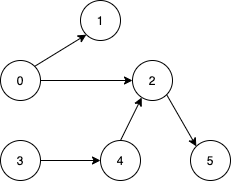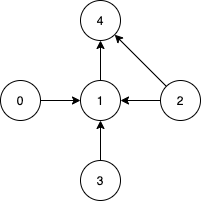1557. Minimum Number of Vertices to Reach All Nodes
Medium
3.3K
111

Given a directed acyclic graph, with `n` vertices numbered from `0` to `n-1`, and an array `edges` where `edges[i] = [fromi, toi]` represents a directed edge from node `fromi` to node `toi`.

Find the smallest set of vertices from which all nodes in the graph are reachable. It's guaranteed that a unique solution exists.

Notice that you can return the vertices in any order.

Example 1:```Input: n = 6, edges = [[0,1],[0,2],[2,5],[3,4],[4,2]]
Output: [0,3]
Explanation: It's not possible to reach all the nodes from a single vertex. From 0 we can reach [0,1,2,5]. From 3 we can reach [3,4,2,5]. So we output [0,3].```

Example 2:```Input: n = 5, edges = [[0,1],[2,1],[3,1],[1,4],[2,4]]
Output: [0,2,3]
Explanation: Notice that vertices 0, 3 and 2 are not reachable from any other node, so we must include them. Also any of these vertices can reach nodes 1 and 4.
```

Constraints:

• `2 <= n <= 10^5`
• `1 <= edges.length <= min(10^5, n * (n - 1) / 2)`
• `edges[i].length == 2`
• `0 <= fromi, toi < n`
• All pairs `(fromi, toi)` are distinct.
Accepted
152.1K
Submissions
186.7K
Acceptance Rate
81.5%

Seen this question in a real interview before?
1/4
Yes
No

Discussion (0)

Related Topics